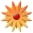# Ratio/proportional Relationships: using graphs, tables, and equations

Page Views: 330

Email This Lesson Plan to Me
Overall Rating:(5.0 stars, 1 ratings)

 Keywords: one step equation, graphs, input output tables, math, proportional relationships Subject(s): Math Grades 6 through 7 NETS-S Standard: Communication and CollaborationCritical Thinking, Problem Solving, and Decision MakingView Full Text of Standards School: Elm Grove Middle School, Bossier City, LA Planned By: Michelle Warehime Original Author: Michelle Warehime, Elm Grove
Lesson Title: Ratio/proportional Relationships: using graphs, tables, and equations
Learner Outcomes: I can relate a ratio tables to graphs and equations.
I can create a graph from a table, a table from an equation,
and a table from a graph.
What students will do: Use equation to make a table and match a graph.
Graph points from a table, make a table from an equation, & use a graph to make a
table.
Higher Level Question: How do you use an equation to check if it is related to a table? A graph?
Activity: Mini match 1 - (Whole class activity, teacher guided) With a given equation, substitute x with a
number from 1-10, solve the equation. Make a table with 3 more ratios. Compare to 2 given tables
and match the table with the
equation. Then match the equation to a graph.
Activity: Graph Match 2 - Students will make ratio tables and coordinate pairs using equations, and match
to graphs in the online game GRAPH MATCH.
http://www1.transum.org/software/graphmatch
Activity: Group Match 3 - Students will use tables, graphs, and equations from previous lessons or ones
they have made from previous lessons. Teacher will collect the one step equations, graphs, and
tables from students previous work and make enough cards for the groups in class.
Students will be given 5 cards of each: tables, graphs, equations and will need to
match 1 of each for five sets. Students will be solving equations: using substitution from the
tables, discussing why the equations match the tables, and then matching the equation and table
to the correct graph.
group or the teacher, why their matches are correct.
Closing: Create a graph from a table, table from an equation, & table from a graph. (Worksheet pre-made
by the teacher. Can be on paper or google forms.)
Assessment: Summative - Answer questions correctly. Explain to their peers how to complete or solve
the work. Tables, Graphs, Equations are done correctly.

Cross-Curricular Science: Ways that you can record data.
Early Finishers: Five extra challenging sets for early finishers.

 Comments This lesson ties in information learned in previous lessons over one-step equations, tables, and graphs. Follow-Up This activity is the follow up to teaching one-step equations, completing an input/output table, and graphing information from equations and tables. Materials: Middle, Math, Power, Projector Screens, Short Throw Projectors, Projectors, Mobile Labs, Games, Word Processor, Student Resources, Assessment Other Items: 1 card stock, \$2.99 each, total of \$2.99